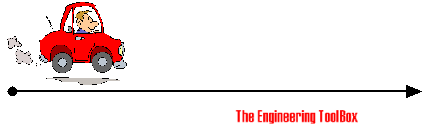Engineering ToolBox - Resources, Tools and Basic Information for Engineering and Design of Technical Applications!

# Average Velocity

## Distance traveled vs. time used.

The average velocity of an object can be expressed as

v = ds / dt                          (1)

where

v = average velocity of object (m/s, ft/s)

ds = distance traveled (m, ft)

dt = time taken (s)

### Example - Average VelocityA car travels a distance of 60 km in one hour. Average velocity can be calculated as

v = (60 km) (1000 m/km)  / ((1 hour) (3600 s/hour))

= 16.7 m/s

= (16.7 m/s) (1/1000 km/m) (3600 s/h)

= 60 km/h

## Related Topics

• ### Dynamics

Motion - velocity and acceleration, forces and torque.

## Related Documents

• ### Acceleration vs. Velocity Equations

Useful equations related to acceleration, average velocity, final velocity and distance traveled.
• ### Piston Engines - Compression Ratios

Cylinder volume and compression ratios in piston engines.
• ### Velocity Units - Online Converter

Convert between common velocity and speed units.
• ### Velocity Units Converter

Convert between speed and velocity units like m/s, km/h, knots, mph and ft/s.

## Engineering ToolBox - SketchUp Extension - Online 3D modeling!

Add standard and customized parametric components - like flange beams, lumbers, piping, stairs and more - to your Sketchup model with the Engineering ToolBox - SketchUp Extension - enabled for use with older versions of the amazing SketchUp Make and the newer "up to date" SketchUp Pro . Add the Engineering ToolBox extension to your SketchUp Make/Pro from the Extension Warehouse !

We don't collect information from our users. More about

## Citation

• The Engineering ToolBox (2008). Average Velocity. [online] Available at: https://www.engineeringtoolbox.com/average-velocity-d_1392.html [Accessed Day Month Year].

Modify the access date according your visit.

9.19.12